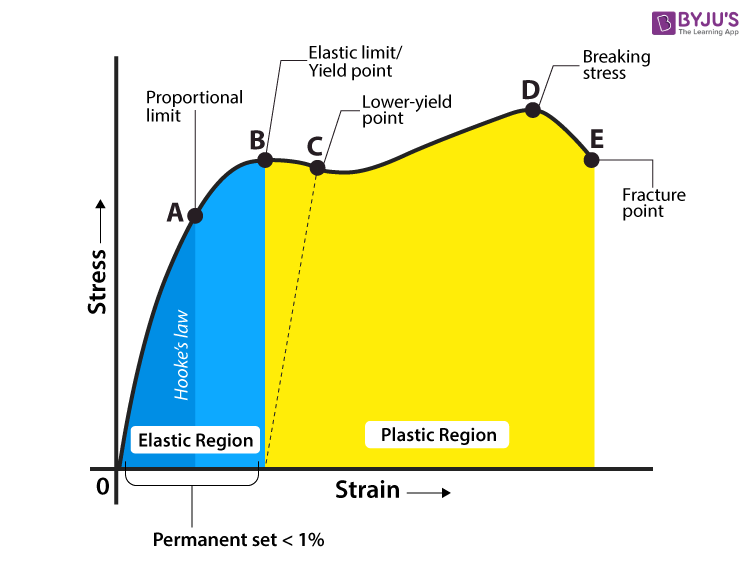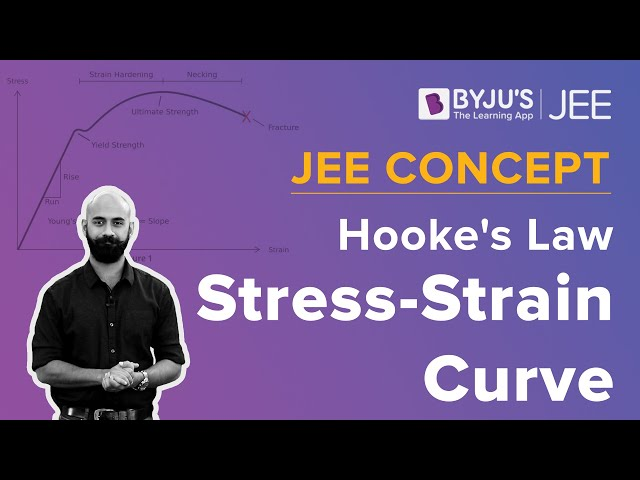# Stress And Strain

You may have noticed that certain objects stretch easily, but stretching objects such as an iron rod sounds impossible, right? In this article, we will help you understand why a few objects are more malleable than others. Mainly, we will discuss Stress-Strain Curve because it help us know the amount of load or stress that a material can handle before it stretches and breaks.

## What is Stress?

Stress is defined as force per unit area within materials that arises from externally applied forces, uneven heating, or permanent deformation and that permits an accurate description and prediction of elastic, plastic, and fluid behaviour.

Stress is given by the following formula:

$$\begin{array}{l}\sigma = \frac{F}{A}\end{array}$$

where, σ is the stress applied, F is the force applied and A is the area of the force application.

The unit of stress is N/m2.

### Types of Stress

Stress applied to a material can be of two types as follows:

#### Tensile Stress

The external force per unit area of the material resulting in the stretch of the material is known as tensile stress.

#### Compressive Stress

Compressive stress is the force that is responsible for the deformation of the material, such that the volume of the material reduces.

## What is Strain?

Strain is the amount of deformation experienced by the body in the direction of force applied, divided by the initial dimensions of the body.

The following equation gives the relation for deformation in terms of the length of a solid:

$$\begin{array}{l}\epsilon = \frac{\delta l}{L}\end{array}$$

where ε is the strain due to the stress applied, δl is the change in length and L is the original length of the material.

The strain is a dimensionless quantity as it just defines the relative change in shape.

### Types of Strain

Strain experienced by a body can be of two types depending on stress application as follows:

#### Tensile Strain

The deformation or elongation of a solid body due to applying a tensile force or stress is known as Tensile strain. In other words, tensile strain is produced when a body increases in length as applied forces try to stretch it.

#### Compressive Strain

Compressive strain is the deformation in a solid due to the application of compressive stress. In other words, compressive strain is produced when a body decreases in length when equal and opposite forces try to compress it.

## Stress-Strain Curve

When we study solids and their mechanical properties, information regarding their elastic properties is most important. We can learn about the elastic properties of materials by studying the stress-strain relationships, under different loads, in these materials.

The material’s stress-strain curve gives its stress-strain relationship. In a stress-strain curve, the stress and its corresponding strain values are plotted. An example of a stress-strain curve is given below.### Explaining Stress-Strain Graph

The different regions in the stress-strain diagram are:

#### (i) Proportional Limit

It is the region in the stress-strain curve that obeys Hooke’s Law. In this limit, the stress-strain ratio gives us a proportionality constant known as Young’s modulus. The point OA in the graph represents the proportional limit.

#### (ii) Elastic Limit

It is the point in the graph up to which the material returns to its original position when the load acting on it is completely removed. Beyond this limit, the material doesn’t return to its original position, and a plastic deformation starts to appear in it.

#### (iii) Yield Point

The yield point is defined as the point at which the material starts to deform plastically. After the yield point is passed, permanent plastic deformation occurs. There are two yield points (i) upper yield point (ii) lower yield point.

#### (iv) Ultimate Stress Point

It is a point that represents the maximum stress that a material can endure before failure. Beyond this point, failure occurs.

#### (v) Fracture or Breaking Point

It is the point in the stress-strain curve at which the failure of the material takes place.

## Hooke’s Law

In the 19th-century, while studying springs and elasticity, English scientist Robert Hooke noticed that many materials exhibited a similar property when the stress-strain relationship was studied. There was a linear region where the force required to stretch the material was proportional to the extension of the material, known as Hooke’s Law.

Hooke’s Law states that the strain of the material is proportional to the applied stress within the elastic limit of that material.

Mathematically, Hooke’s law is commonly expressed as:

F = –k.x

Where F is the force, x is the extension in length, and k is the constant of proportionality known as the spring constant in N/m.

See the video below to learn about the stress-strain curve and Hooke’s law.## Elastic Moduli of Materials

The following table lists Young’s modulus, shear modulus and bulk modulus for common materials.

 Material Young’s modulus (E) in GPa Shear modulus (G) in GPa Bulk modulus (K) in GPa Glass 55 23 37 Steel 200 84 160 Iron 91 70 100 Lead 16 5.6 7.7 Aluminium 70 24 70

Note: GPa is gigapascal and 1 GPa = 1,00,00,00,000 Pa.

## Frequently Asked Questions – FAQs

Q1

### What does the stress-strain curve show?

A stress-strain curve is a graphical way to show the reaction of a material when a load is applied. It shows a comparison between stress and strain.
Q2

### What is the use of the stress-strain diagram?

The stress-strain diagram provides a graphical measurement of the strength and elasticity of the material. Also, the behaviour of the materials can be studied with the help of the stress-strain diagram, which makes it easy to understand the application of these materials.
Q3

### What is the relation between stress and strain?

The relation between stress and strain is that they are directly proportional to each other up to an elastic limit. Hooke’s law explains the relationship between stress and strain. According to Hooke’s law, the strain in a solid is proportional to the applied stress and this should be within the elastic limit of that solid.
Q4

### What is the yield point in stress-strain graph?

Yield point is defined as the point at which the material starts to deform plastically. After the yield point is passed, permanent plastic deformation occurs.
Q5

### How to make a stress-strain curve?

The stress-strain curve is obtained by gradually applying load to a test coupon and measuring the deformation, from which the stress and strain can be determined.

Stay tuned to BYJU’S and Fall in Love with Learning!

Test your knowledge on Stress And Strain